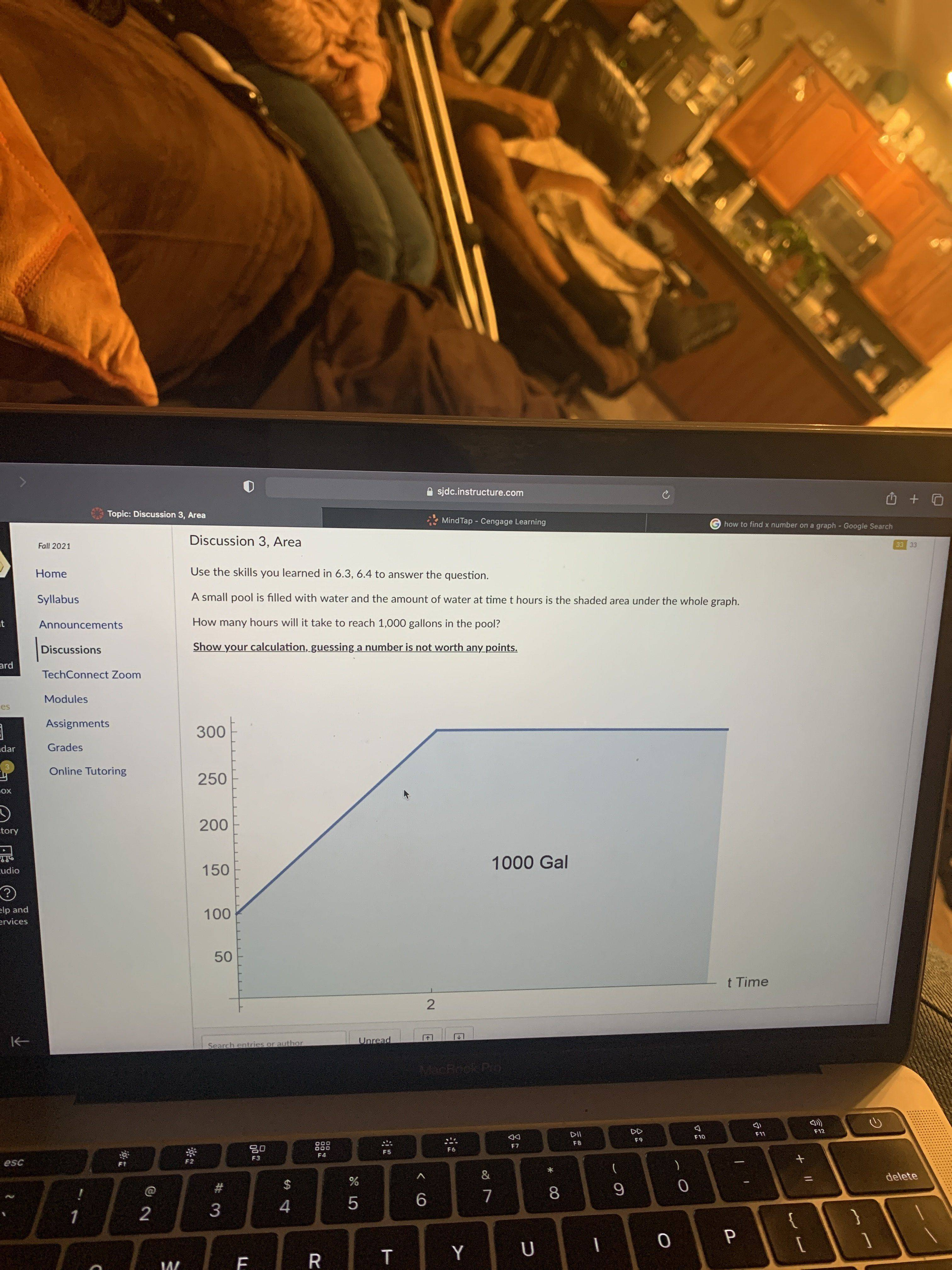# Time to fill water tank

• MHB
sophbellGold Member
MHB
The area represented under the curve is a triangle (up to t = 2 hours) plus a rectangle. How do you find the sum of that area?

-Dan

HOI
Draw a horizontal line at "100" all the way across. Draw a vertical line at "2" all the way up and down. That divides the area into a right triangle with one leg of length 2 and the other of length 200, a rectangle with width 2 and height 100, and another rectangle with height 300 and unknown width. What is the area of the triangle and the first rectangle?

If that is less than 1000, subtract if from 1000. How wide must the second rectangle be so that its area is that difference?

Gold Member
MHB
The area represented under the curve is a triangle (up to t = 2 hours) plus a rectangle. How do you find the sum of that area?

-Dan

Surely it's a trapezium and a rectangle...